# Solving PDE's with chebychev FFT

• I
I have seen one lecturer solve a PDE with just using Fast Fourier Transform (##FFT##) of a function ##v## on a chebychev grid. ##v_t=\mu v_x##

This lecturer uses ##FFT## on ##V##, then solves the ODE using an ODE solver in Matlab, then inverse ##FFT## to get the real solution :
$$FFT(v_t)=\hat{v_t}=FFT(\mu v_x)\\ =>\frac{\partial{\hat{v}}}{\partial{t}}=iK\mu \hat{v}$$
##K## is a vector of wave numbers, ##FFT## is fast fourier transform of a function, ##ifft## is the inverse fast fourier transform, and ##i## is the imaginary number.

the lecturer solves for ##\hat{v}## with an ODE solver, then inverse fourier transform to get solution ##v##.

BUT, in the book Spectral Methods for Matlab, the author first transforms the function with ##FFT##, then he calculates the derivative in Fourier space, then the author claims he must transform this back into ##x## space to get the derivative. So, $$\mu \hat{v}_x=\mu iK\hat{v}\\ v_x=-\frac{ifft(\mu iK\hat{v})}{\sqrt{1-x^2}}$$

the ##\sqrt{1-x^2}## comes from chain rule derivative, where the author states when he takes the ##FFT## of the function, it puts the function into ##\theta## space, so to get the x derivative the author has to use chain rule for some reason.

I can't figure out why the methods are different. The only idea I have is maybe the first lecturer's function V is approximately zero at the boundaries, while the Spectral Methods for Matlab author's function is not zero at the boundaries. Could this be the only difference?

Delta2
Homework Helper
Gold Member
I understand what the lecturer does , and that is standard theory of transforming PDEs to ODEs using fourier transform.

However I can't quite understand what the book does, and how exactly it uses the chain rule. Can you post screenshots of the respective book pages?

I understand what the lecturer does , and that is standard theory of transforming PDEs to ODEs using fourier transform.

However I can't quite understand what the book does, and how exactly it uses the chain rule. Can you post screenshots of the respective book pages?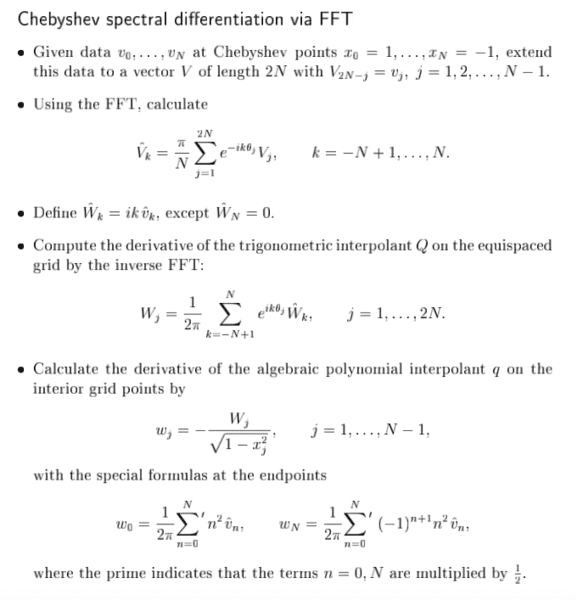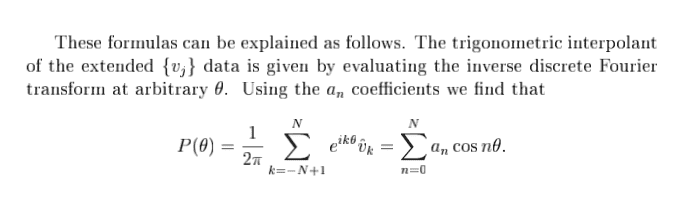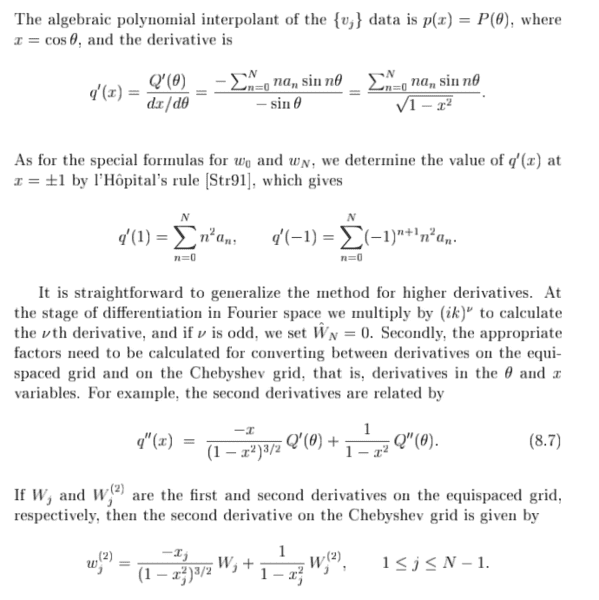This function outputs the derivative of a grid function v based on the above method :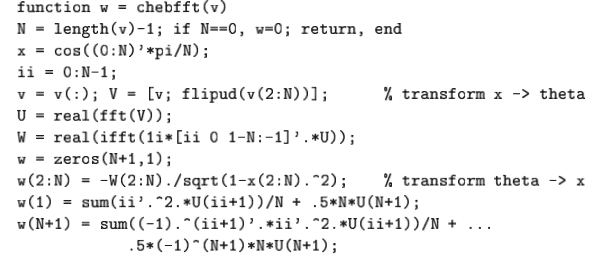#### Attachments

Delta2
Homework Helper
Gold Member
I have trouble understanding what the book does (especially the part with the algebraic and trigonometric interpolant of the given data), but I think at the end it calculates the derivative of a known function (given data). It doesn't solve a PDE.

I have trouble understanding what the book does (especially the part with the algebraic and trigonometric interpolant of the given data), but I think at the end it calculates the derivative of a known function (given data). It doesn't solve a PDE.

Yeah, I know, but it is used to solve a pde, ##u_{tt}=u_{xx}##. Author uses leap from in time and chebfft (the program above) twice to approximate the x derivative.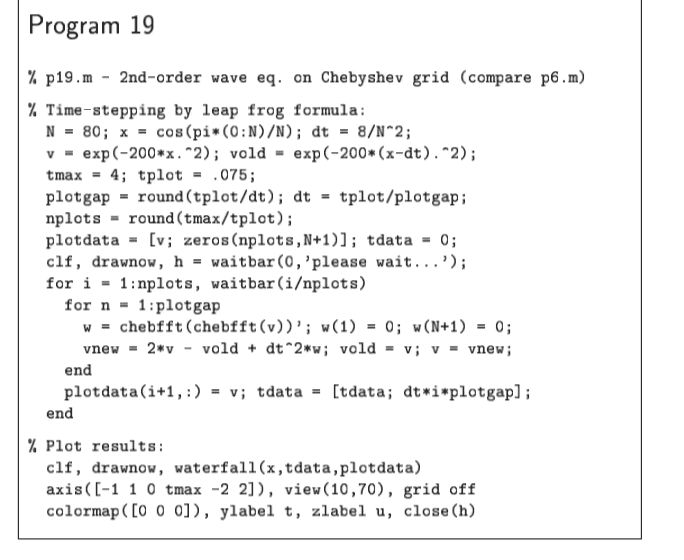#### Attachments

Delta2
Homework Helper
Gold Member
Well the book in calculating the derivative for some reason it chooses to work in ##\theta## space (I guess for better accuracy), that is it first does the transform ##x=\cos\theta## and then it calculates ##v'(\theta)## with the aid of FFT, and then it uses the chain rule to calculate for ##v'(x)=v'(\theta)\frac{d\theta}{dx}=v'(\theta)\frac{1}{\sqrt{1-x^2}}##(1).

It doesn't solve a PDE which would involve ##\theta## and ##x## as independent variables.

I guess you interpret equation (1) as some sort of PDE, no it isn't a PDE.

Well the book in calculating the derivative for some reason it chooses to work in ##\theta## space (I guess for better accuracy), that is it first does the transform ##x=\cos\theta## and then it calculates ##v'(\theta)## with the aid of FFT, and then it uses the chain rule to calculate for ##v'(x)=v'(\theta)\frac{d\theta}{dx}=v'(\theta)\frac{1}{\sqrt{1-x^2}}##(1).

It doesn't solve a PDE which would involve ##\theta## and ##x## as independent variables.

I guess you interpret equation (1) as some sort of PDE, no it isn't a PDE.

The program in my last post, program 19, is solving $$\frac{\partial^2 u}{\partial t^2} = \frac{\partial^2 u}{\partial x^2}$$ That is the PDE I am talking about. The author is solving it with leapfrog in ##t## and fft on chebyshev points in ##x##, but the only difference seems to be the ##\sqrt{1-x^2}## term. I don't understand why one author needs this extra term and the other doesn't. Why one author considers it ##\theta## space and the other does not.

The only answer I can think of is maybe the initial condition is zero at the boundaries in one case and not zero in the other case.

Delta2
Homework Helper
Gold Member
The program in my last post, program 19, is solving $$\frac{\partial^2 u}{\partial t^2} = \frac{\partial^2 u}{\partial x^2}$$ That is the PDE I am talking about. The author is solving it with leapfrog in ##t## and fft on chebyshev points in ##x##, but the only difference seems to be the ##\sqrt{1-x^2}## term. I don't understand why one author needs this extra term and the other doesn't. Why one author considers it ##\theta## space and the other does not.

The only answer I can think of is maybe the initial condition is zero at the boundaries in one case and not zero in the other case.
I am not sure either why the book works with the FFT and iFFT in ##\theta## space (it might had to do with the boundary conditions or for better accuracy in the calculation of the derivative), but that's where the extra term ##\sqrt{1-x^2}## comes from.

The lecturer works in all cases with FFT and iFFT in x-space. The book, inside chebfft(v(x)) works with FFT and iFFT in ##\theta## space not in x-space. so it calculates ##v_{\theta}=iFFT(\mu iK\hat{v})## and then it uses the chain rule ##v_x=v_{\theta}\cdot\frac{d\theta}{dx}##. When ##x=\cos\theta## or equivalently ##\theta=\arccos x## then ##\frac{d\theta}{dx}=\frac{-1}{\sqrt{1-x^2}}##.

I am not sure either why the book works with the FFT and iFFT in ##\theta## space (it might had to do with the boundary conditions or for better accuracy in the calculation of the derivative), but that's where the extra term ##\sqrt{1-x^2}## comes from.

The lecturer works in all cases with FFT and iFFT in x-space. The book, inside chebfft(v(x)) works with FFT and iFFT in ##\theta## space not in x-space. so it calculates ##v_{\theta}=iFFT(\mu iK\hat{v})## and then it uses the chain rule ##v_x=v_{\theta}\cdot\frac{d\theta}{dx}##. When ##x=\cos\theta## or equivalently ##\theta=\arccos x## then ##\frac{d\theta}{dx}=\frac{-1}{\sqrt{1-x^2}}##.

So, if ##iFFT## is in ##\theta##-space, and the author transforms the answer back into ##x##-space, than doesn't that mean the author has to initially transform the data from ##x##-space to ##\theta##-space? But the author starts out with a function defined on a chebyshev grid, and just uses chebfft on it. I don't see where the author originally transforms it from ##x##-space to ##\theta##-space, so I don't understand why he is transforming it back.

Delta2
Homework Helper
Gold Member
So, if ##iFFT## is in ##\theta##-space, and the author transforms the answer back into ##x##-space, than doesn't that mean the author has to initially transform the data from ##x##-space to ##\theta##-space?

I think it does the transform of data from the x-space to θ - space in the lines from 2 to 4 inside the function chebfft(v), at least that's what I see in the image you uploaded and contains the code for chebfft(v). Inside it defines a new V that contains the transformed data and then it does FFT to V (not to v) , and proceeds with iFFT on the result it gets.

•fahraynk
I think it does the transform of data from the x-space to θ - space in the lines from 2 to 4 inside the function chebfft(v), at least that's what I see in the image you uploaded and contains the code for chebfft(v). Inside it defines a new V that contains the transformed data and then it does FFT to V (not to v) , and proceeds with iFFT on the result it gets.
Thanks again,

$$v=v(:)\\ V=[v;flipud(v(2:N))];$$

I thought those 2 lines just makes the grid function periodic, although I admit I don't fully understand why he did this!

If I take those lines out and just set : $$w=iFFT(i*K*FFT(v))$$, do you think it would be the same solution? I wonder why this "transform" makes the equation more accurate when he is already using chebychev grid points, what good does it do making it periodic with some weird flipping function (flipud)...

•Delta2
Delta2
Homework Helper
Gold Member
It is a mystery to me too why it choses to work in θ -space .

If you take out those lines and proceed as you say, I don't think you ll get exactly the same solution but approximately the same solution maybe. You will also have to remove the line that applies the chain rule (line 8 or 9), otherwise you ll get totally different solutions.